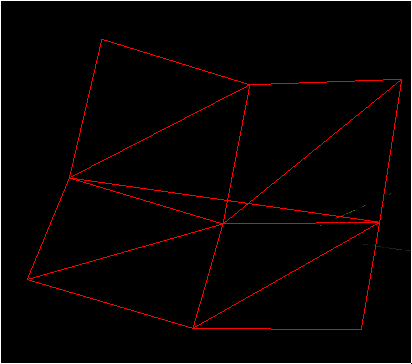# Paraboloid-shaped terrain has superfluous line in wireframe mode

Hi,

Based on triangle strips (inspired by a tutorial from leanopengles(dot)com), I’m drawing a 3x3 terrain where positions of vertexes belong to a paraboloid (3D parabola).

I did add duplicated two vertexes at the end of the row (to produce degenerate triangles) and everything seemed ok when rendering, except there is a line at the middle of the mesh in wireframe mode (see image below).

The list of indices I got is: 0,3,1,4,2,5,5,3,3,6,4,7,5,8 (vertexes in bold are duplicates) if you start counting from the top left, and line-by-line.
I suspect it’s one of the degenerate triangles but doesn’t OpenGL ignore triangles with a null area?

PS: the number of elements I pass to `glDrawElements()` is the number of indices above (incl. duplicated indices). And for a flat terrain, there’s no superfluous line or triangle like in the picture attached.If you rasterise a null-area triangle as a triangle, the number of fragments inside that triangle is zero and nothing will be rendered. If you rasterise its edges as lines, they will be visible (you’ll get two identical lines and one zero-length line).

Basically, you can’t use degenerate triangles in conjunction with `glPolygonMode(GL_LINE)`, because that trick only works for filled triangles.

Either:

• Use `GL_TRIANGLES` instead of `GL_TRIANGLE_STRIP`
• Use `GL_TRIANGLE_STRIP` for filled triangles but `GL_LINES` (with a different index array) for lines
• Use `glEnable(GL_PRIMITIVE_RESTART)` and `glPrimitiveRestartIndex` instead of degenerate triangles (requires OpenGL 3.1 or later).